NEET  >  Test: Ideal Gas Equation & Dalton's Law of Partial Pressure

# Test: Ideal Gas Equation & Dalton's Law of Partial Pressure

Test Description

## 16 Questions MCQ Test Chemistry Class 11 | Test: Ideal Gas Equation & Dalton's Law of Partial Pressure

Test: Ideal Gas Equation & Dalton's Law of Partial Pressure for NEET 2023 is part of Chemistry Class 11 preparation. The Test: Ideal Gas Equation & Dalton's Law of Partial Pressure questions and answers have been prepared according to the NEET exam syllabus.The Test: Ideal Gas Equation & Dalton's Law of Partial Pressure MCQs are made for NEET 2023 Exam. Find important definitions, questions, notes, meanings, examples, exercises, MCQs and online tests for Test: Ideal Gas Equation & Dalton's Law of Partial Pressure below.
Solutions of Test: Ideal Gas Equation & Dalton's Law of Partial Pressure questions in English are available as part of our Chemistry Class 11 for NEET & Test: Ideal Gas Equation & Dalton's Law of Partial Pressure solutions in Hindi for Chemistry Class 11 course. Download more important topics, notes, lectures and mock test series for NEET Exam by signing up for free. Attempt Test: Ideal Gas Equation & Dalton's Law of Partial Pressure | 16 questions in 16 minutes | Mock test for NEET preparation | Free important questions MCQ to study Chemistry Class 11 for NEET Exam | Download free PDF with solutions
 1 Crore+ students have signed up on EduRev. Have you?
Test: Ideal Gas Equation & Dalton's Law of Partial Pressure - Question 1

### Direction (Q. Nos. 1-12) This section contains 12 multiple choice questions. Each question has four choices (a), (b), (c) and (d), out of which ONLY ONE option is correct. Q.  The vapour pressure of liquid water is 23.8 torr at 25°C. By what factor does the molar volume of water increases as it vaporises to form an ideal gas under these conditions? The density of liquid water is 0.997 g cm-3 at 298 K ?

Test: Ideal Gas Equation & Dalton's Law of Partial Pressure - Question 2

### A gas at a pressure of 5.0 bar is heated from 0°C to 546°C and is simultaneously compressed to one-third of its original volume. Hence, final pressure is

Detailed Solution for Test: Ideal Gas Equation & Dalton's Law of Partial Pressure - Question 2

P1V1/T1 = P2V2/T2
Let the original volume be 3 volume units . The final volume will be 1/3 of 3 which is 1 volume unit
Temperature must be in K - 0 DEG C = 273K and 546 DEG C = 819 K
We want to solve for P1
P1*1/819 = 5*3/273
P1 = 819*5*3/273
P1 = 45

Test: Ideal Gas Equation & Dalton's Law of Partial Pressure - Question 3

### A 8.3 L cylinder contains 128 . 0 g O2 gas at 27°C. What mass of O2 gas must be released to reduce the pressure to 6.0 bar ?

Detailed Solution for Test: Ideal Gas Equation & Dalton's Law of Partial Pressure - Question 3

By applying ideal gas eqn. PV = nRT
Further solving it we get an eq.
w = PVM/RT
Putting values, we get 64g.
Hence, the correct answer is Option A.

Test: Ideal Gas Equation & Dalton's Law of Partial Pressure - Question 4

N2 gas under the given state I is to be changed to state II. Thus, N2 escaped from I is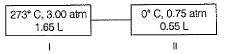Detailed Solution for Test: Ideal Gas Equation & Dalton's Law of Partial Pressure - Question 4

Applying pV = nRT in state 1, we have n1 = 0.110
Applying pV = nRT in state 1, we have n2 = 0.0184
Mole fraction(this fraction is present) = 0.0184/0.110 = 0.16727
So, fraction escaped = (1-0.16727)*100 = 83.33%

Test: Ideal Gas Equation & Dalton's Law of Partial Pressure - Question 5

1 g H2 gas and x g O2 gas exert a total pressure of 5 atm. At a given temperature partial pressure of O2 gas is 4 atm. This O2 in the mixture is

Test: Ideal Gas Equation & Dalton's Law of Partial Pressure - Question 6

The average molar mass of the vapour above solid NH4CI is nearly 26.75 g mol-1.

Thus, vapour consists of (mole fraction)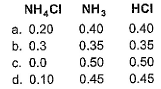Detailed Solution for Test: Ideal Gas Equation & Dalton's Law of Partial Pressure - Question 6

The correct answer is Option C.

The average molar mass of the vapour above solid NH4Cl = 26.5 mol-1
Evaporation product of NH4Cl --->
NH4Cl (s) ----> NH3(g) + HCl(g)
Let Mole fraction of NH3 = XL
Mole fraction of HCl = X2
∴ Average molar mass of NH3 = 14 + 31 = 17
=> 26.5 = m1x1 + m2x2
=> 26.5 = 17x1 + 36.5x2    ---(i)
And we know;
x1 + x2 = 1
From (i) and (ii)
x1 = 0.5 and x2 = 0.5

Test: Ideal Gas Equation & Dalton's Law of Partial Pressure - Question 7

Pressure of 1 g of an ideal gas A at 300 K is found to be 2 bar. When 2 g of another ideal gas B is introduced in the same flask at the same temperature, pressure becomes 3 bar. Thus,

Detailed Solution for Test: Ideal Gas Equation & Dalton's Law of Partial Pressure - Question 7

Given pressure(P) of gas A= 2bar Total pressure = 3bar then pressure(P) of gas B = 3-2 =1bar
no. of mole (n) = given mass(m) / molar mass(M)
Gas A
PV = nRT
2×V =( mₐ/Mₐ) RT ------------- (1)
Gas B
PV = nRT
1V = (mb/Mb)RT ---------------- (2)
On dividing eqn 1 by eqn 2
2V/1V = (1/Mₐ) ÷(2/Mb)
2/1 = (Mb/Mₐ) × 1/2
(MB/Mₐ) = (2/1)×(2/1)
MB/Mₐ = 4/1
MB = 4Mₐ

Test: Ideal Gas Equation & Dalton's Law of Partial Pressure - Question 8

Total pressure of a mixture of H2 and O2 is 1.00 bar. The mixture is allowed to react to form water, which is completely removed to leave only pure l-l2 at a pressure of 0.35 bar. Assuming ideal gas behaviour and that all pressure measurements were made under the same temperature and volume conditions, the composition of the original mixture is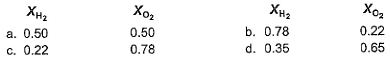Detailed Solution for Test: Ideal Gas Equation & Dalton's Law of Partial Pressure - Question 8

Equal volume of two gases at same pressure and temperature conditions have equal number of molecules.
∴1 O2 molecule consumes 2 H2 molecules.
∴Since after the reaction only H2 is left,  we know all of O2 is consumed.
∴This implies what all consumed 1/3 of it was O2.
Hence, O2 in the mix was at a partial pressure of (1 - 0.35)* 1/3 = 0.22
And rest was H2 = (1 - 0.22) = 0.78
Hence oxygen- hydrogen composition in the original mix was:0.22 bar O2 and 0.78 bar H2.

Test: Ideal Gas Equation & Dalton's Law of Partial Pressure - Question 9

The composition of air inhaled by a human is 21 % by volume O2 and 0.03% CO2 and that of exhaled air is 16% O2 and 4.03% CO2. Assuming a typical volume of 6200 L day -1, moles of O2 used and CO2 generated by the body at 37°C and 1.00 bar are (assume ideal behaviour)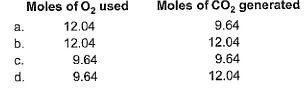Test: Ideal Gas Equation & Dalton's Law of Partial Pressure - Question 10

The following arrangement of flasks is set up. Assuming no temperature change, determine the final pressure inside the system after all stopcocks are opened. The connecting tube has zero volume.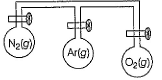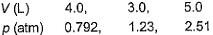Detailed Solution for Test: Ideal Gas Equation & Dalton's Law of Partial Pressure - Question 10

Applying p1V1 + p2V2 + p3V3 = pnet × Vnet
(0.792)×4 + (1.23)×3 + (2.51)×5 = pnet × 12
On solving, we get p = 1.617 = 1.62 atm

Test: Ideal Gas Equation & Dalton's Law of Partial Pressure - Question 11

1.00 mole sample of O2 and 3.00 moles sample of H2 are mixed isothermally in a 125.3 L container at 125°C. Gaseous mixture undergoes reaction to form water vapours. Assume ideal gas behaviour total pressure before and after H2O (g) is formed is are respectivel

Detailed Solution for Test: Ideal Gas Equation & Dalton's Law of Partial Pressure - Question 11

Correct option is not provided.
2H2     +    O2 ------>   2H2O
3         1                0
(-)   2         1                2 mole H2O formed
___________________________
1               0              Total mole = 1+2 = 3

PV = nRT / 100
n = PV / RT
P = nRT/ V
= [4 * 0.083 * (125 + 273)] / 125.3
Pressure = 1.0545 bar after water formed is 2 mole.
P = [3 * 0.083 * 398] /125.3
= 0.7909 bar
= 0.791 bar (appx)
Hence, the answer is 1.0545 bar, 0.7909 bar.

Test: Ideal Gas Equation & Dalton's Law of Partial Pressure - Question 12

When NO2 is cooled to room temperature, some of it reacts to form a dimer N2O4 through the reaction,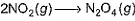In a reaction, 15.2 g of NO2 is placed in a 10.0 L flask at high temperature and the flask is cooled to 25°C. The total pressure is measured to be 0.500 atm. Thus, partial pressure of NO2 is found to be

Detailed Solution for Test: Ideal Gas Equation & Dalton's Law of Partial Pressure - Question 12

nNO2 + 2nN2O4= 0.330 mol
Subtracting nNO2+ nN2O4 = 0.204 mol
From nNO2+ 2nN2O4 = 0.330 mol
nNO2 = 0.126 mol
nN2O4 = 0.078 mol
From these results, NO2 has a mole fraction of 0.62and a partial pressure of 0.62(0.50 atm) = 0.31 atm;N2O4has a mole fraction of 0.38 and a partial pressure of 0.38(0.50 atm) = 0.19 atm

*Answer can only contain numeric values
Test: Ideal Gas Equation & Dalton's Law of Partial Pressure - Question 13

Direction (Q. Nos. 13 and 16) This section contains 4 questions. when worked out will result in an integer from 0 to 9 (both inclusive)

Q.
A toy balloon originally held 1.00 g helium gas and had a radius of 10.0 cm.
During the night 0.25 g of the gas effused from the balloon. Assuming the ideal gas behaviour under the constant pressure and temperature conditions, what was the radius of the balloon the next morning? (Express result in cm.)

Detailed Solution for Test: Ideal Gas Equation & Dalton's Law of Partial Pressure - Question 13

We know that
pV = nRT
A/Q , p and T are constant;
So  n1/n2 = V1/V2
(¼)/(0.25/4) = (10)3/r3
r3 = 750
r  ∼ 9

*Answer can only contain numeric values
Test: Ideal Gas Equation & Dalton's Law of Partial Pressure - Question 14

What will be the temperature difference needed in a hot air balloon to lift 1.00 kg of mass ? Assume that the volume of the balloon is 100 m3, the temperature of the ambient air is 298 K, the pressure is 1.00 bar, and the air is an ideal gas with average molar mass of 29 g mol-1.

Detailed Solution for Test: Ideal Gas Equation & Dalton's Law of Partial Pressure - Question 14

The solution is as follows:
Δm = mcold − mhot
=[ncold−nhot]M
= PVMR[1T1− 1T2]
Solving we get:
T2−T1 = ΔT = 2

*Answer can only contain numeric values
Test: Ideal Gas Equation & Dalton's Law of Partial Pressure - Question 15

Iron forms a series of compounds of the type Fex (CO)y. In air, they are oxidised to Fe2O3 and CO2 gas. After heating a 0.142 g sample in air, you isolate the CO2 in a 1.50 L flask at 25°C. The pressure of the gas is 44.9 mm Fig. What will be the value of y/x  in the simplest empirical formula of Fex(CO)y? [Atomic mass of Fe = 56]

Detailed Solution for Test: Ideal Gas Equation & Dalton's Law of Partial Pressure - Question 15

The empirical formula is = Fe(CO)2
Carbon dioxide obtained:
Pressure = 44.9 mm Hg
Also, P (mm Hg) = P (atm) / 760
Pressure = 44.9 / 760 = 0.0591 atm
Temperature = 25 °C
The conversion of T( °C) to T(K) is shown below:
T(K) = T( °C) + 273.15
So,
T₁ = (25 + 273.15) K = 298.15 K
V = 1.50 L
Using ideal gas equation as:
PV=nRT
where,
P is the pressure
V is the volume
n is the number of moles
T is the temperature
R is Gas constant having value
= 0.0821 L.atm/K.mol
Applying the equation as:
0.0591 atm × 1.50 L
= n × 0.0821 L.atm/K.mol × 298.15 K
⇒n = 0.0036 moles
1 mole of carbon atoms are present in 1 mole of carbon dioxide. So,
Moles of C = 0.0036 moles
Molar mass of C atom
= 12.0107 g/mol
Mass of C in molecule
= 0.0036 x 12.0107 = 0.0432 g
Given that the compound only contains iron and carbon. So,
Mass of Fe in the sample
= Total mass - Mass of C
Mass of the sample = 0.142 g
Mass of Fe in sample
= 0.142 g - 0.0432 g = 0.0988 g
Molar mass of Fe = 55.845 g/mol
Moles of Fe
= 0.0988  / 55.845  = 0.0018 moles
Taking the simplest ratio for Fe and C as:
0.0018 : 0.0036
= 1 : 2

*Answer can only contain numeric values
Test: Ideal Gas Equation & Dalton's Law of Partial Pressure - Question 16

To an evacuated vessel with movable piston under external pressure of 1 atm, 0.1 mole of Fie and 0.1 mole of an unknown compound (vapour pressure 0.68 atm at 0°C) are introduced. Considering the ideal gas behaviour, the total volume (in litre) of the gases 0°C is close to...

[IITJEE 2011]

Detailed Solution for Test: Ideal Gas Equation & Dalton's Law of Partial Pressure - Question 16

Let nx mole of unknown compound be present in vapour form so its partial pressure is
0.68 = (nx/nx+0.1)×1 atm
nx+0.1/nx = 1/0.68 = 1.47
0.1/nx = 0.47
nx = 0.212
Applying ideal gas equation,
1×V = (0.212/0.1)×0.0821×273
V = 7

## Chemistry Class 11

199 videos|330 docs|229 tests
Information about Test: Ideal Gas Equation & Dalton's Law of Partial Pressure Page
In this test you can find the Exam questions for Test: Ideal Gas Equation & Dalton's Law of Partial Pressure solved & explained in the simplest way possible. Besides giving Questions and answers for Test: Ideal Gas Equation & Dalton's Law of Partial Pressure, EduRev gives you an ample number of Online tests for practice

## Chemistry Class 11

199 videos|330 docs|229 tests

### How to Prepare for NEET

Read our guide to prepare for NEET which is created by Toppers & the best Teachers(Scan QR code)